Year:The 2016 Canadian Open Mathematics Challenge — Nov 3/4Nicolae Strungaru

## Problem of the Week

by Assoc. Prof. Nicolae Strungaru, Grant MacEwan University

The COMC has three parts. In part A solutions do not require work be shown and may be possible to do in your head. In part B the problems begin to draw on more knowledge and have some more challenging aspects that will need a pencil and paper to solve. By Part C the problems require that work be shown and involve arguments to support the answer.

We have selected a cross section of contest problems from a variety of national and regional contests that we hope will stimulate interest in problem solving and give some experience to get ready to write the Canadian Open Mathematics Competition in November. The problem areas are not tied to particular grade levels, or to the curriculum but cover a number of areas from algebra, through logic and some geometry.

We will post solutions to these problems one week later, but teachers should be aware that determined students may be able to locate solutions elsewhere online before then.

For a more comprehensive set of problems and solutions at each of these levels, please feel welcome to download past official exams and solutions from our archive.

Week 9

• Problem (posted November 1st)

Find all polynomials $f(x)=x^n+a_{1}x^{n-1}+a_2x^{n-2}+...+a_{n-1}x+a_n$ with the following properties:

1. all the coefficients $a_1,..,a_{n}$ belong to the set $\{ -1,1\}$;
2. all the roots of the equation $f(x)=0$ are real.

• Solution (posted November 8th)

This was Problem 5 of the Fourth Irish Mathematical Olympiad, 1991, which appeared in Crux Mathematicorum [1993:192-193]. We present the solution by Michael Selby which appeared at [1995:12-13].

Let $r_1,r_2,..,r_n$ represent the roots of this polynomial. Then \begin{align} r_1+r_2+...+r_n &= -a_{1} \cr r_1r_2+r_1r_3+...+r_{n-1}r_n &= a_{2} \cr r_1r_2...r_n &= (-1)^na_n \,. \end{align}

We therefore get \begin{align} r_1^2+r_2^2+..+r_n^2 &= \left(r_1+...+r_n\right)^2-2 \left(r_1r_2+r_1r_3+...+r_{n-1}r_n \right) \cr &= 1-2a_{2} \,. \end{align}

This implies that, as long as $n \geq 2$, we have $1-2a_2 \geq 0$ and hence $a_2=-1$.

Next, by the Arithmetic Mean-Geometric Mean inequality we get \begin{align} \frac{r_1^2+...+r_n^2}{n} &\geq \sqrt[n]{r_1^2r_2^2...r_n^2} \cr &= \sqrt[n]{ \left( (-1)^na_n \right)^2}=1 \end{align} with equality if and only if $r_1^2=r_2^2=...=r_n^2=1$.

Therefore $1-2a_2 \geq n \,,$ with equality if and only if $r_1^2=r_2^2=...=r_n^2=1$.

Therefore $n \leq 3$.

Case 1: $n=1$. It is straightforward to see that in this case both $X+1$ and $X-1$ work.

Case 2: $n=2$. Then we must have $a_2=-1$, therefore, the possible polynomials are $X^2-X-1$ and $X^2+X-1$, and both work.

Case 3: $n=3$. Then we have $a_2=-1$ and equality in the AM-GM inequality, hence $r_j \in \{ \pm 1 \}$. Moreover, since $r_1r_2+r_1r_3+r_2r_3=-1$, it is not possible for all three roots to have the same sign. This leaves us with two possible polynomials: \begin{align} (X-1)^2(X+1) &= X^3-X^2-X+1 \cr (X-1)(X+1)^2 &= X^3+X^2-X-1 \cr \end{align}

Answer: There are 6 such polynomials: $X-1 \,;\, X+1 \,;\, X^2-X-1 \,;\, X^2+X-1 \,;\, X^3-X^2-X+1 \mbox{ and } X^3+X^2-X-1 \,.$

Week 8

• Problem (posted October 25th)

We cut an equilateral triangle $AXY$ from the rectangle $ABCD$ in such a way that the vertex $X$ is on side $BC$ and that vertex $Y$ is on side $CD$. Prove that among the remaining three right triangles there are two, the sum of whose areas equals the area of the third.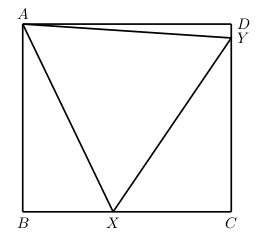• Solution (posted November 1st)

This was Problem 2 of the Hungarian National Olympiad, 1987, which appeared in Crux Mathematicorum [1989:100-101]. We present the solution by Michael Selby and D.J. Smeenk which appeared at [1991:68-69].

First, this is not possible in every rectangle. If the rectangle has sides $a \leq b$, then a necessary condition is that $a \geq \frac{\sqrt{3}}{2}b$.

Now, if we can cut such a triange, let $s$ denote the side of the triangle. We claim that $[XYC]=[ADY]+[ABX] \,,$ where $[T]$ denotes the area of the triangle $T$. Let us denote by $\theta_1$ and $\theta_2$, respectively the angles $\angle BAX$ and $\angle DAY$, respectively.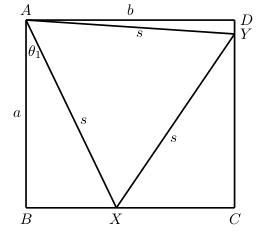Then \begin{align} [ABX] &= \frac{1}{2} AB \cdot BX \cr &= \frac{1}{2} s \cos(\theta_1) s \sin(\theta_1) \cr &= \frac{1}{2} s^2 \cos(\theta_1) \sin(\theta_1) \cr &= \frac{1}{4} s^2 \sin(2 \theta_1) \,, \end{align} and same way $[ADY] =\frac{1}{4} s^2 \sin(2 \theta_2) \,.$

Therefore \begin{align} [ADY] &= \frac{1}{4} s^2 \sin(2 \theta_2) \cr &= \frac{1}{4} s^2 \sin\left(2 (30^\circ -\theta_1)\right) \cr &= \frac{1}{4} s^2 \sin\left(60^\circ -2\theta_1\right) \cr &= \frac{1}{4} s^2 \left( \frac{\sqrt{3}}{2} \cos(2\theta_1) -\frac{1}{2} \sin(2\theta_1) \right) \cr &= \left(\frac{\sqrt{3}}{8} s^2 \cos(2\theta_1) \right) -\left( \frac{1}{8} s^2 \sin(2\theta_1) \right) \,. \end{align}

This shows that $[ADY]+[ABX]= \frac{\sqrt{3}}{8} s^2 \cos(2\theta_1) +\frac{1}{8} s^2 \sin(2\theta_1) \,.$

Now, for $XCY$ the angle $\angle CXY= 30^\circ+\theta_1$. Therefore \begin{align} [CXY] &= \frac{1}{2} CX \cdot CY \cr &= \frac{1}{2} s^2 \sin(30^\circ+\theta_1) \cos(30^\circ+\theta_1) \cr &= \frac{1}{4} s^2 \sin(60^\circ+2 \theta_1) \cr &= \frac{\sqrt{3}}{8} s^2 \cos(2\theta_1) +\frac{1}{8} s^2 \sin(2\theta_1) \cr &= [ADY]+[ABX] \,. \end{align}

Week 7

• Problem (posted October 18th)

$ABC$ is a triangle with $AB=AC$ and $\angle BAC=20^\circ$. On the side $AC$ we pick inside a point $D$ such that $AD=BC$. Find the measure of the angle $\angle CDB$.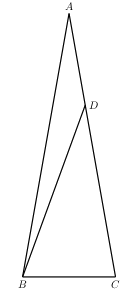• Solution (posted October 25th)

This is one of my favourite geometry problems, with a very nice solution. Unfortunately we lost the source of this problem and of the solution below.

On side $AD$ we construct outside an equilateral triangle $ADE$. Connect $E$ to $B$.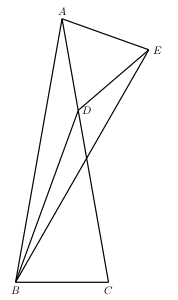Now, by SAS, triangles $ABC$ and $BAE$ are congruent. Indeed $AB=AC \,;\, \angle ABC=80^\circ =\angle BAE \,;\, BC=AE \,.$

Then, it follows that triangle $BAE$ is isosceles and hence $AB=BE$.

Then, by SSS, triangles $ADB$ and $EDB$ are congruent. Indeed $AD=ED \,;\, DB=DB \,;\, AB=EB \,.$ It follows that $\angle ADB=\angle EDB$.

Since $\angle ADB+\angle EDB+60^\circ =360^\circ$ we get $\angle ADB =150^\circ \,.$ Then $\angle CDB =30^\circ \,.$

Week 6

• Problem (posted October 11th)

Find all the natural numbers $n$ such that the number $n(n+1)(n+2)(n+3)$ has exactly 3 prime divisors.

• Solution (posted October 18th)

Problem 5 of the 30th Spanish Mathematical Olympiad, Final Round, 1993, which appeared in Crux Mathematicorum [1998:69-70]. We present the solution by Edward T.H. Wang which appeared at [1999:203-204], slightly modified.

We prove that the only such integers are $n=2,3$ and $6$.

Let $P(n)=n(n+1)(n+2)(n+3)$. Then $P(1)=2^3 \cdot 3$ and thus $n=1$ is not a solution. Hence we assume $n \geq 2$.

Note first that for all $k \in \mathbb{N}$ we have $(k,k+1)=(2k-1,2k+1)=1$.

We have two cases:

Case 1: $n$ is odd. Then the numbers $n, n+1, n+2$ are by the above pairwise relatively prime, and hence each needs to be a power of a prime.

Since $n+1$ is even, we must have $n=p^a \,;\, n+1=2^b \, \mbox{ and } \, n+2=q^c$ where $a, b, c, p, q \in \mathbb N$ with $p,q$ distinct odd primes.

Note now that $n+3=2^{b}+2=2(2^{b-1}+1)$ where $b \geq 2$. Since the only possible prime divisors of $n+3$ are $2, p$ and $q$ and $(n+2,n+3)=1$ we must have $2^{b-1}+1=p^d \,,$ with $d \geq 1$.

Therefore, $2p^d=n+3=p^a+3 \,.$

This shows that $p|3$ and hence $p=3$. Dividing by $3$, we get $2 \cdot 3^{d-1}=3^{a-1}+1 \,.$

Now, we cannot have $d-1>0$ and $a-1>0$, as in this case we would get $3|1$. Therefore one of $d-1$ or $a-1$ must be $0$, and it is immediate that the other one is also zero.

This shows that $a=1$, and hence $n=3$.

We showed that the only possible solution with $n$ odd is $n=3$, and it is easy to check that this is indeed a solution.

Case 2: $n$ is even. Then, we have $(n+1,n+2)=(n+1,n+3)=(n+2,n+3)=1$. By the same argument as in Case 1 we must have $n+1=p^a \,;\, n+2=2^b \, \mbox{ and } \, n+3=q^c$ where $a, b, c, p, q \in \mathbb N$ with $p,q$ distinct odd primes.

Now, $n=2^b-2=2(2^{b-1}-1) \,.$

We know that $n\geq 2$ and hence $b \geq 2$.

If $b=2$ we get $n=2$ which is a solution.

Otherwise $b \geq 3$ and hence $2^{b-1}-1$ is an odd number greater or equal than $3$. The only primes which can divide $n$ are $2,p,q$ and as $(n, p^a)=1$ and $2^{b-1}-1$ is odd, we must have $2^{b-1}-1=q^d \,,$ with $d \geq 1$.

Therefore, $2q^d=n=q^c-3 \,.$ This shows that $q|3$ and hence $q=3$. Dividing by $3$, we get $2 \cdot 3^{d-1}=3^{c-1}-1 \,.$ We must have $d-1 \leq c-1$ and hence $3^{d-1} |1$. This shows that $d=1$ and hence $n=2 \cdot 3=6 \,.$

It is easy to check that $n=6$ is indeed a solution.

In conclusion, $n(n+1)(n+2)(n+3)$ has exactly three prime divisors if and only if $n \in \{2,3,6\}$.

Week 5

• Problem (posted October 4th)

This week we look at a functional equation.

Find all functions $f : \mathbb{N}^* \to \mathbb{N}^*$ such that $f\left(f(m)+f(n) \right) = m+n \,;\, \forall m,n \in \mathbb{N}^* \,,$ where $\mathbb{N}^*$ denotes the set $\{1,2,3,...\}$ of positive integers.

• Solution (posted October 11th)

Problem 4 of the 44th Lithuanian Mathematical Olympiad, which appeared in Crux Mathematicorum [1998:196-197]. We present the solution by Pierre Bornsztein which appeared at [1999:334-335].

For all $m,n \in \mathbb{N}^*$, we have $f\left(f(m)+f(n) \right) = m+n \tag{1}$ $f\left(f(m)+f(n) \right)+f\left(f(m)+f(n) \right) =2( m+n) \,.$

Therefore \begin{align} f\left[ f\left( f(m)+f(n) \right)+f\left(f(m)+f(n) \right) \right] & = f(m)+f(n)+f(m)+f(n) \cr & = 2\left( f(m)+f(n) \right) \end{align} and $f\left[ f\left(f(m)+f(n) \right)+f\left(f(m)+f(n) \right) \right] = f(2m+2n) \,.$

It follows that $f(2m+2n)=2\left( f(m)+f(n) \right) \,. \tag{2}$

Setting $m=n$, we get $4f(n)=f(4n)$.

Setting $m=2p+1, n=2p-1$ we also get $2f(2p+1)+2f(2p-1)=f(8p)=4f(2p)$ for all $p \geq 1$, and hence $f(2p+1)=2f(2p)-f(2p-1) \,. \tag{3}$

Setting $m=2p+2, n=2p-2, (p \geq 2)$ we get $f(2p+2)=2f(2p)-f(2p-2) \,. \tag{4}$

Let us set $f(1)=a, f(2)=b$.

Using $(3)$ and $(4)$ we get \begin{align} f(3) &= 2b-a \cr f(4) &= f(4 \cdot 1)=4f(1)=4a \cr f(5) &= 9a-2b \cr f(6) &= 8a-b \end{align}

Now, using $m=2,n=1$ in $(2)$ we get $f(6)=2f(2)+2f(1)=2a+2b \,.$

Thus $8a-b=2a+2b,$ hence $b=2a$. It follows that for $n \in \{ 1, 2, 3, 4, 5, 6 \}$ we have $f(n)=an \,.$

Now, using $(3)$ and $(4)$ by induction we get immediately $f(n)=an, \, \mbox{ for all } n \in \mathbb{N}^*.$

Now, by $(1)$, for all $m,n \in \mathbb{N}^*$ we have $m+n=f\left(f(m)+f(n) \right)=a(f(m)+f(n))=a^2(m+n) \,.$

Therefore $a=1$ and $f(n)=n \, \mbox{ for all } n \in \mathbb{N}^* \,.$

Conversely, $f(n)=n$ works.

Week 4

• Problem (posted September 27th)

This week we will look at an inscribed circle problem.

Let $ABC$ be an equilateral triangle and $\Gamma$ its incircle. If $D$ and $E$ are points on the sides $AB$ and $AC$, respectively, such that $DE$ is tangent to $\Gamma$, show that $\frac{AD}{DB}+\frac{AE}{EC}=1$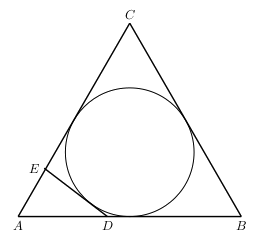• Solution (posted October 4th)

Problem 4 of of the 8th IberoAmerican Mathematical Olympiad 1993, which appeared in Crux Mathematicorum [1996:159-160]. We present the solution by Sěfket Arslanagić which appeared at [1997:465-466].

Let $AB=AC=BC=a, BD=p$ and $CE=q$. Then $AD=a-p$ and $AE=a-q$.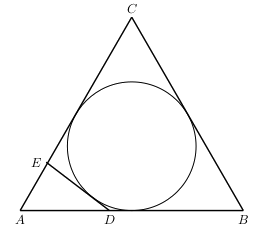Since the circle $\Gamma$ is inscribed in the quadrilateral $BCED$ we have $ED+BC=BD+CE \,,$ or $ED=p+q-a \,.\tag{1}$

By the Law of cosines in the triangle $ADE$ we get $ED^2=AD^2+AE^2-2AD \cdot AE \cdot \cos(60^\circ) \,,$ and hence, by $(1)$ $(p+q-a)^2=(a-p)^2+(a-q)^2-(a-p)(a-q) \,.$ This gives $3pq=ap+aq\,.$

Then \begin{align} \frac{AD}{DB}+\frac{AE}{EC} & = \frac{a-p}{p}+\frac{a-q}{q} \cr & = \frac{aq-pq+ap-qp}{pq} \cr & = \frac{ap+aq-2pq}{pq} \cr & = 1 \,. \end{align}

Week 3

• Problem (posted September 20th)

Let $p$ be the number of functions defined on the set $\{ 1, 2, 3, ..., m \}$, $m \in \mathbb{N}^{*}$, with values in the set $\{1, 2, ..., 35, 36\}$ and $q$ be the number of functions defined on the set $\{ 1, 2, 3, ..., n \}$, $n \in \mathbb{N}^{*}$, with values in the set $\{1, 2, 3, 4, 5\}$. Find the least possible value for the expression $|p-q|$.

• Solution (posted September 27th)

Problem 5 of the Republic of Moldova XL Mathematical Olympiad, form 11, which appeared in Crux Mathematicorum [1999:326-327]. We present the solution by Pierre Bornsztein which appeared at [2001:369-370], slightly modified.

Let $m,n \in \mathbb{N}^{*}$. We have $p=36^m$ and $q=5^n$. The problem is then to find the least possible value of $|36^m-5^n|$ over all $m,n \in \mathbb{N}^{*}$.

The last two digits of $36^m$ can be $36, 96, 56, 16$ or $76$. The last two digits of $5^n$ are either $5$ or $25$.

This shows that $36^m-5^n \in \{ \pm 9, \pm 11, \pm 29, \pm 31, \pm 49 \} \pmod{100}$

Let us also observe that since $9|36^m$ and $9 \nmid 5^n$ we have $9 \nmid 36^m-5m$. Therefore $36^m-5^n \neq \pm 9$.

This shows that the smallest possible value $|36^m-5^n|$ could take is $11$. This value is achieved when $m=n=2$.

Therefore, the least possible value of $|p-q|$ is 11.

Week 2

• Problem (posted September 13th)

This week we look at a Quintic Equation.

Find all real solutions to the equation $1+x+x^2+x^3=x^4+x^5 \,.$

• Solution (posted September 20th)

Problem 4 of the $21^{st}$ W.J. Blundon Mathematics Contest, Memorial University which was written in February 2004 and given in the Skoliad Corner of Crux Mathematicorum in [2005:354].

The equation can be written as $(1+x)(1+x^2)=(1+x)x^4 \,.$

It is clear that $x=-1$ is a solution.

If $x \neq -1$ we can divide by $1+x$ to get $1+x^2=x^4 \,,$ or $x^4-x^2-1=0 \,.$

Substituting $y=x^2$ we get the quadratic equation $y^2-y-1=0$ with solutions $y_1= \frac{1+\sqrt{5}}{2} \mbox{ and } y_2= \frac{1-\sqrt{5}}{2} \,.$

Since $y_2 <0$ the equation $x^2=y_2$ has no solution.

Solving $x^2=y_1$ we get two more real solutions $x= \pm \sqrt{\frac{1+\sqrt{5}}{2}} \,.$

Therefore, the equation has $3$ real solutions: $x=-1, x=\sqrt{\frac{1+\sqrt{5}}{2}} \mbox{ and } x=-\sqrt{\frac{1+\sqrt{5}}{2}} \,.$

Week 1

• Problem (posted September 6th)

We give two entry level problems this week. Give them a try. Look for the source and the solution next week!

Problem A
In the diagram, $ABCD$ is a square with side length 17 and the four triangles $ABF, DAE, BCG$, and $CDH$ are congruent right triangles. Furthermore, $\overline{FB}=8$. Find the area of the shaded quadrilateral $EFGH$.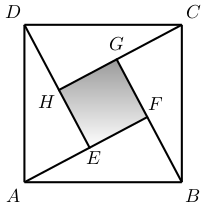Problem B
Find the number of solutions in integers $(x,y)$ of the equation $x^2y^3=6^{12} \,.$

• Solution (posted September 13th)

Problem A

This was Problem 1 of BC Colleges High School Mathematics Contest 2005, Junior Final Round B, written in May 2005, and given in the Skoliad Corner of Crux Mathematicorum in [2005:265]. We give the "official" solution which appeared at [2006:137].

Since $FB=8$ and $AB=17$, the Pythagorean Theorem gives $AF=15$. The area of each of the four congruent triangles is $\frac{8 \times 15}{2}=60$. Thus the area of the shaded square $EFGH$ is $17^2-4\cdot 60=49$.

Problem B

This was Problem 3 of the same contest. We give the "official" solution which appeared at [2006:138].

First, since $6^{12} >0$ and $x^2 \geq 0$ we must have $x^2 >0$ and $y>0$.

We are given that $x^2y^3=6^{12}=2^{12}3^{12}$. Since $x$ and $y$ both divide $2^{12}3^{12}$ we must have $x= \pm 2^i3^j$ and $y= 2^k3^l$, where $i,j,k$ and $l$ are non-negative integers.

Then $x^2=2^{2i}3^{2j}$ and $y^3=2^{3k}3^{3l}$. Therefore $x^2y^3=2^{2i+3k}3^{2j+3l} \,.$

We want $x^2y^3=2^{12}3^{12}$. Thus $2i+3k=12$ and $2j+3l=12$. Solving these equations we get $(i,k) \in \{ (0,4), (3,2), (6,0) \} \mbox{ and } (j,l) \in \{ (0,4), (3,2), (6,0) \} \,.$

Now, each of the three values of $i$ can be paired with each of the three values of $j$. Once this is done, the values of $k$ and $l$ are determined. Therefore, the number of solutions in positive integers is $3 \times 3=9$, and the the total number of solutions is $9 \times 2=18$.